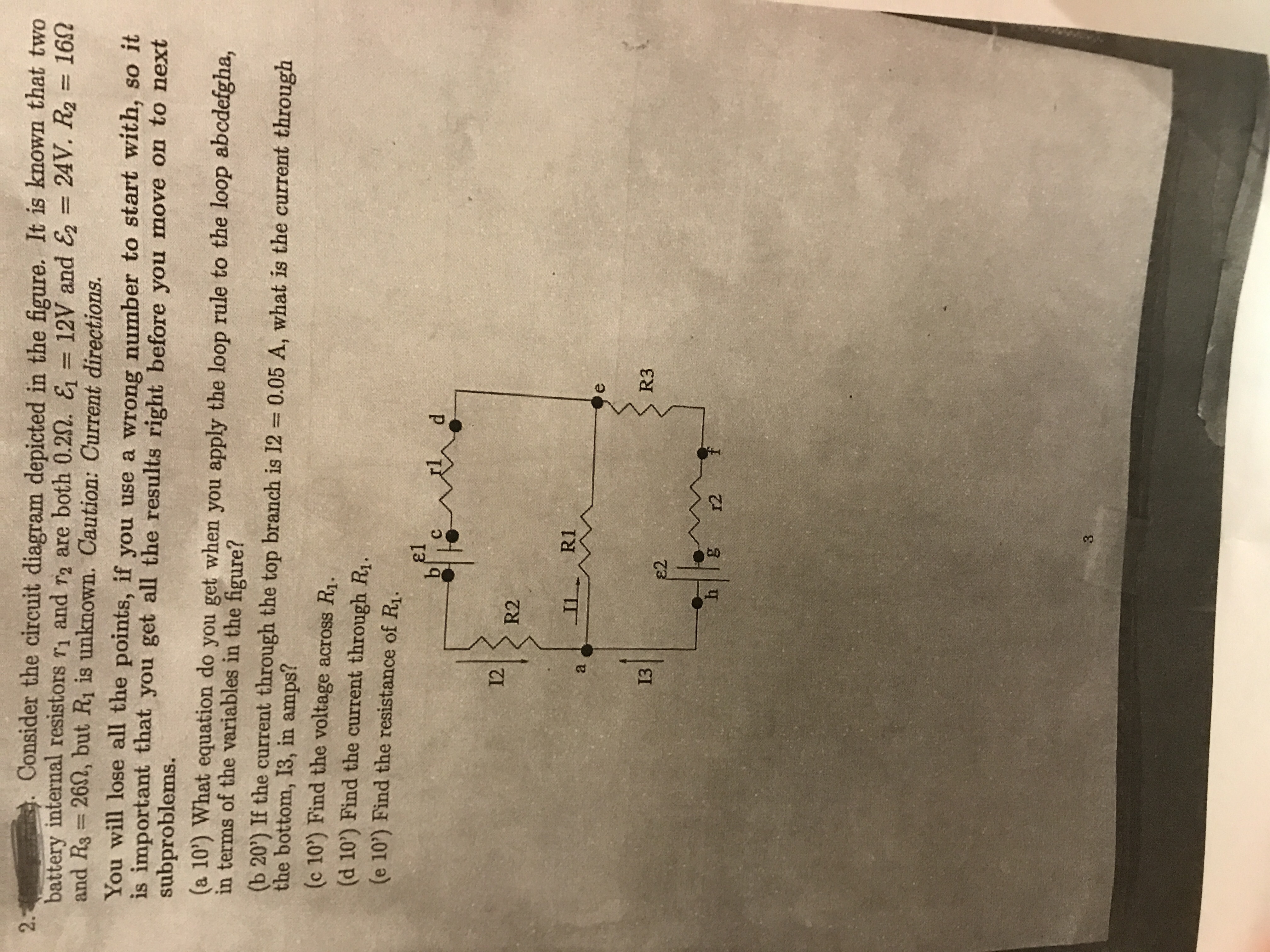Consider the circuit diagram depicted in the fgure. It is known that twobattery internal resistors r1and r2 are both 0.2Ω· = 12V and & = 24V. R2 16Ω2.and Rs 262, but Ri is unknown. Caution: Current directions.You will lose all the points, if you use a wrong number to start with, so iis important that you get all the results right before you move on to n,subproblems(a 10) What equation do you get when you apply the loop rule to the loop abcdefgha,in terms of the variables in the figure?b 20) If the current through the top branch is 12 0.05 A, what is the current throughthe bottom, 13, in amps?(c 10) Find the voltage across R1.(d 10') Find the current through Ri.(e 10) Find the resistance of Ri.el12 R2R3132h g123

Questionhelp_outlineImage TranscriptioncloseConsider the circuit diagram depicted in the fgure. It is known that two battery internal resistors r1and r2 are both 0.2Ω· = 12V and & = 24V. R2 16Ω 2. and Rs 262, but Ri is unknown. Caution: Current directions. You will lose all the points, if you use a wrong number to start with, so i is important that you get all the results right before you move on to n , subproblems (a 10) What equation do you get when you apply the loop rule to the loop abcdefgha, in terms of the variables in the figure? b 20) If the current through the top branch is 12 0.05 A, what is the current through the bottom, 13, in amps? (c 10) Find the voltage across R1. (d 10') Find the current through Ri. (e 10) Find the resistance of Ri. el 12 R2 R3 13 2 h g12 3 fullscreen
Step 1

Given,

Battery Internal resistance, r1 = r1 = 0.2 Ω

e.m.f of batteries, ε1 = 12 V and ε2 = 24 V

Resistance, R2 = 16 Ω and R3 = 26 Ω

Step 2

Apply Kirchhoff’s 2nd law in loop abcdefgha.

Step 3

If current I2 = 0....

Want to see the full answer?

See Solution

Want to see this answer and more?

Our solutions are written by experts, many with advanced degrees, and available 24/7

See Solution
Tagged in

Current Electricity